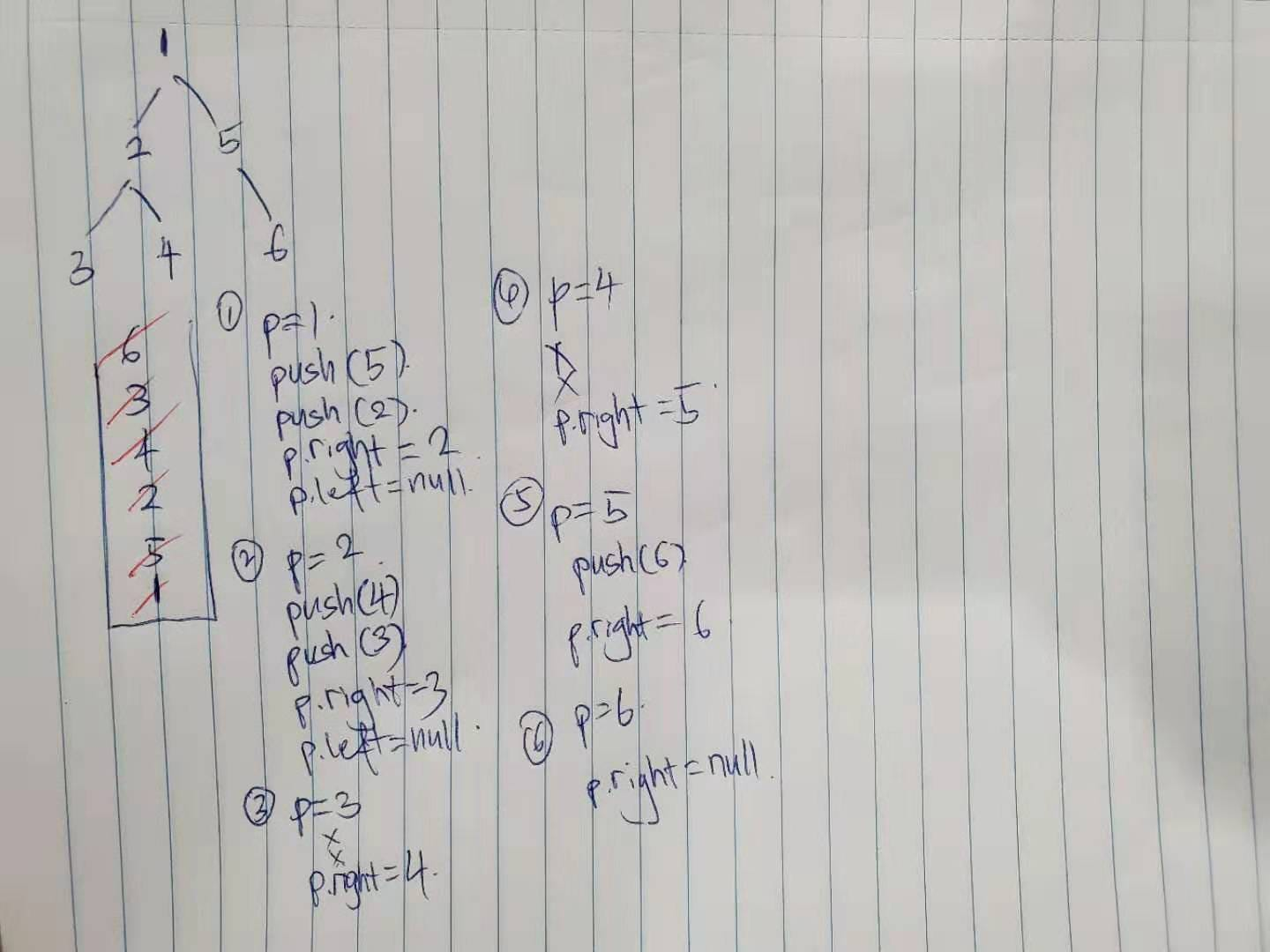# 114. Flatten Binary Tree to Linked List

Reference: LeetCode
Difficulty: Medium

## Problem

Given a binary tree, flatten it to a linked list in-place.

Example:

The flattened tree should look like:

## Analysis

### Preorder

The idea is to use preorder traversal. For example, when root = 1, we want to set 1.right as 2 and set 4.right as 5.

Notice that to know where 4 is during the traversal, we can use a prev node to keep track of the previous node. The previous node of 1 is 4.

Note: I know the code could be shorter, but I think shorter code is barely readable!

Time: $O(N)$
Space: $O(h)$

### Recursive Function

Note: Just a bit modification on the previous solution.

This idea is a more recursive idea. Let’s say we have a flatten() function. For node 1, we can flatten its two children 2 and 5. At that time, we don’t need to know the detail but we are sure that we will have:

Two subproblems have been flattened.

Time: $O(N)$
Space: $O(N)$

### LeetCode Solution

More like reversed postorder!

I don’t like this following method neither.

Other Solution: (not using field variable)

Time: $O(N)$
Space: $O(h)$

### Iteration (Preorder)

Use a stack to simulate the preorder traversal, but we also use peek operation to set current node’s right child to its left child.Note:

• Before peeking, remember to check if the stack is empty.
• Actually, we don’t have to check the stack’s size before calling peek(). If the stack is empty, it will just return null. But it does not hold true for Stack‘s peek().

Time: $O(N)$
Space: $O(h)$

CommentJunhao Wang
a software engineering cat